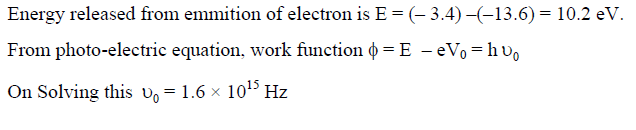Question 1

A current loop consists of two identical semicircular parts each of radius R, one lying in the x-y plane and the other in x-z plane. If the current in the loop is i. The resultantmagnetic field due to the two semicircular parts at their common centre is

A : µ0i / 2√2 R
B : µ0i / 2R
C : µ0i / 4R
D : µ0i / √2R

•
.

Solution :
B = √(Bxy2 + Bxz2)
= √((µ0i / 4R)2 + (µ0i / 4R)2)
0i√2 / 4R)2
0i / 2√2 R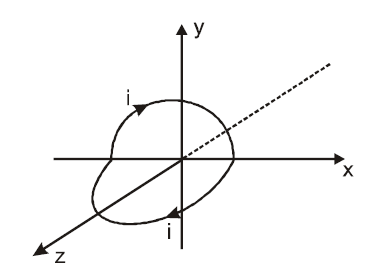•   Question 2

Two following figure shows a logic gate circuit with two inputs A and B and the output Y. the voltage wave forms of A, B and Y are as given.
The logic gate is:?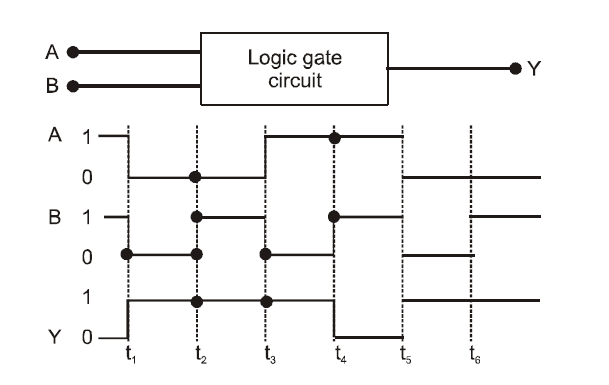A : NOR
B : OR
C : AND
D : NAND

•
.

Solution :
It is clear from given logic circuit, that output Y is low when both the inputs are high, otherwise it is high. Thus logic circuit is NAND gate.

•   Question 3

Two parallel metal plates having charges +Q and -?Q face each other at a certain distance between them. If the plates are now dipped in kerosene oil tank, the electric field between the plates will

A : become zero
B : increase
C : decrease
D : remains same

•
.

Solution :
Electric field between two parallel plates placed in vacuum is given by
E = σ/ ε0
In a medium of dielectric constant K,
E'= σ / ε0K
For kerosene oil K > 1
so E' = E

•   Question 4

The electric field at a distance 3R/2 from the centre of a charged conducting spherical shell of radius R is E. The electric field at a distance R/2 from the centre of the sphere is

A : 0
B : E
C : E/2
D : E/3

•
.

Solution :
Electric field inside charged conductor is always zero.

•   Question 5

Astudent measures the distance traversed in free fall of abody, initially at rest, in a given time. He uses this data to estimate g, the acceleration due to gravity. If themaximum percentage errors in measurement of the distance and the time are e1 and e2 respectively, the percentage error in the estimation of g is

A : e2 - e1
B : e1 + 2e2
C : e1 + e2
D : e1 - 2e2

•
.

Solution :
using h= ut + 1/2gt2
we get Δg/g =Δh/h -2Δt/t
For max permissible error
(Δg/g *100) =(Δh/h *100 ) +2*(Δt/t *100)
Δh/h *100 = e1
Δt/t *100 = e2
therefore (Δg/g *100) = e1 + 2e2

•   Question 6

Whenmonochromatic radiation of intensity ? falls on ametal surface, the number of photoelectrons and their maximum kinetic energy are N and T respectively. If the intensity of radiation is 2?, the number of emitted electrons and their maximum kinetic energy are respectively

A : N and 2T
B : 2N and T
C : 2N and 2T
D : N and T

•
.

Solution :
The number of photoelectrons ejected is directly proportional to the intensity of incident light. Maximum kinetic energy is independent of intensity of incident light but depends upon the frequency of light. Hence option (2) is correct.

•   Question 7

The electric field of an electromagnetic wave in free space is given by Ê = 10cos(107t + kx)ĵ V/m, where t and x are in seconds and metres respectively. It can be inferred that
(i) the wavelength λ is 188.4 m.
(ii) the wave number k is 0.33 rad/m
(iii) the wave amplitude is 10 V/m(iv) the wave is propagating along +x direction
Which one of the following pairs of statements is correct ?

A : iii and iv
B : i and ii
C : ii and iii
D : i and iii

•
.

Solution :
Comparing it with standard equation of e.m. wave,
E= E0cos(wt + kx)
c = vλ = wλ/2π
λ= 2πc /w ;
λ=188.4m
also c=w/k
k=w/c = 0.033

•   Question 8

The speed of light in media M1 and M2 are 1.5 � 108 m/s and 2.0 � 108 m/s respectively.A ray of light enters from medium M1 to M2 at an incidence angle i. If the ray suffers total internal reflection, the value of i is:

A : Equal to sin-(2/3)
B : Equal to or less than sin-(3/5)
C : Equal to or greater than sin-(3/4)
D : Less than sin-(2/3)

•
.

Solution :
µ1 = c/v1 =2
µ2 = c/v2 =3/2
For TIR sin i ≥ sin C
sin C= µ2 / µ1 sin i ≥ µ21 ≥ (3/2)/2
Equal to or greater than sin-(3/4)

•   Question 9

A ray of light is incident on a 60° prism at the minimum deviation position. The angle of refraction at the first face (i.e., incident face) of the prism is

A : 0
B : 30°
C : 45°
D : 60°

•
.

Solution :
Angle of prism, A = r1 + r2 For minimum deviation r1 = r2 = r
A = 2r
Given, A= 60°
so r = A/2 =30°

•   Question 10

For transistor action
(1) Base, emitter and collector regions should have similar size and doping concentrations.
(2) The base region must be very thin and lightly doped.
(3) The emitter-base junction is forward biased and base-collector junction is reverse biased
(4) Both the emitter-base junction as well as the base-collector junction are forward biased

A : 4 and 1
B : 1 and 2
C : 2 and 3
D : 3 and 4

•
.

•   Question 11

The additional kinetic energy to be provided to a satellite of mass m revolving around a planet of mass M, to transfer it from a circular orbit of radius R1 to another of radius R2 (R2 > R1) is

A : GmM(1/R12 - 1/R22 )
B : GmM(1/R1 - 1/R2 )
C : 2GmM(1/R1 - 1/R2 )
D : 1/2 *GmM(1/R1 - 1/R2 )

•
.

Solution :
KE = (-GmM/2R2) - (-GmM/2R1)

•   Question 12

The speed of a projectile at its maximum height is half of its initial speed. The angle of projection is(in deg)

A : 60
B : 15
C : 45
D : 60

•
.

Solution :
v= ucosΘ
given v = u/2
u/2 = ucosΘ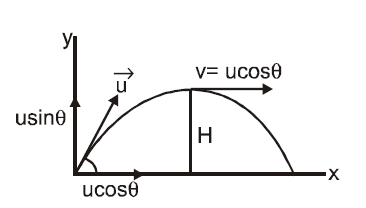•   Question 13

Froma circular disc of radius Randmass 9M, a small disc ofmassMand radius R/3 is removed concentrically. The moment of inertia of the remaining disc about an axis perpendicular to the plane of the disc and passing through its centre is

A : 40/9 * MR2
B : MR2
C : 4MR2
D : 4/9 * MR2

•
.

Solution :
Themoment of inertia of the complete disc about an axis passing through its centre O and perpendicular to its plane is
I1 = 9/2 * MR2
I2 = 1/18 * MR2
I = I1 - I2

•   Question 14

A particle moves in x-y plane according to rule x = asin wt and y = acos wt. The particle follows

A : an elliptical path
B : a circular path
C : a parabolic path
D : a straight line path inclined equally to x and y-axes

•
.

Solution :
x/a = sin wt
\ y/a = cos wt
square and add the 2 equations and get equation of circle

•   Question 15

A closely wound solenoid of 2000 turns and area of cross-section 1.5 * 10-?4 m2 carries a current of 2.0 A. It is suspended through its centre and perpendicular to its length, allowing it to turn in a horizontal plane in a uniform magnetic field 5 * 10?-?2 tesla making an angle of 30° with the axis of the solenoid. The torque on the solenoid will be

A : 3 * 10 -3 Nm
B : 1.5 * 10 -3 Nm
C : 1.5 * 10 -2 Nm
D : 3 * 10 -2 Nm

•
.

Solution :
Magnetic moment of the loop.
M = NIA = 2000 * 2 * 1.5 * 10-?4 = 0.6 J/T
torque t = MBsin30°

•   Question 16

The decay constant of a radio isotope is λ. If A1 and A2 are its activities at times t1 and t2 respectively, the number of nuclei which have decayed during the time (t1 ? t2)

A : A1t1 - A2t2
B : A1 - A2
C : (A1 - A2) /λ
D : λ/(A1 - A2)

•
.

Solution :
(A1 = λN1 at t1
(A2 = λN2 at t2
Therefore, number of nuclei decayed during time interval (t1-t2) is
N1 - N2 =[A1-A2]/λ

•   Question 17

A particle having a mass of 10-?2 kg carries a charge of 5 * 10?-8 C. The particle is given an initial horizontal velocity of 105 ms?-?1 in the presence of electric field E and magnetic field B. To keep the particle moving in a horizontal direction, it is necessary that
(i) B should be perpendicular to the direction of velocity and E should be along the direction of velocity (ii) Both B and E should be along the direction of velocity
(iii) Both B and E are mutually perpendicular and perpendicular to the direction of velocity.
(iv) B should be along the direction of velocity and E should be perpendicular to the direction of velocity
Which one of the following pairs of statements is possible

A : i and iii
B : iii and iv
C : ii and iii
D : ii and iv

•
.

•   Question 18

The binding energy per nucleon in deuterium and helium nuclei are 1.1 MeV and 7.0 MeV, respectively. When two deuterium nuclei fuse to form a helium nucleus the energy released in the fusion is

A : 23.6MeV
B : 2.2MeV
C : 28.0MeV
D : 30.2MeV

•
.

Solution :
The binding energy per nucleon of a deuteron = 1.1 MeV
so Total binding energy = 2 * 1.1 = 2.2 MeV
so The binding energy per nucleon of a helium nuclei = 7 MeV
so Total binding energy = 4 * 7 = 28 MeV
so Hence, energy released
ΔE = (28 ? 2 * 2.2) = 23.6 MeV

•   Question 19

The electron in the hydrogen atom jumps from excited state (n = 3) to its ground state (n = 1) and the photons thus emitted irradiate a photosensitive material. If the work function of the material is 5.1 eV, the stopping potential is estimated to be (the energy of the electron in nth state En = -13.6/n2 eV

A : 5.1 V
B : 12.1V
C : 17.2V
D : 7V

•
.

Solution :
Energy released when electron in the atom jumps from excited state (n = 3) to ground state (n = 1) is
E = hv
E3 - E1 = 12.1eV
therefore stopping potential
eV0 = hv - φ
=12.1-5.1
V0 = 7V

•   Question 20

If cp and cv denote the specific heats (per unit mass) of an ideal gas of molecular weight M where R is the molecular weight constant, then

A : cp-cv = R/M2
B : cp - cv = R
C : cp - cv =R/M
D : cp - cv = MR

•
.

Solution :
Let CV and CP be molar specific heats of the ideal gas at constant volume and constant pressure, respectively, then
CP = Mcp and CV = Mcv
CP-CV = R
Mcp - Mcv = R
cp - cv =R/M

•   Question 21

A condenser of capacity C is charged to a potential difference of V1. The plates of the condenser are then connected to an ideal inductor of inductance L. The current through the inductor when the potential difference across the condenser reduces to V2 is

A : ( (C(V1 - V2)2)/L )1/2
B : C(V12 - V22)/L
C : C(V12 - V22)/L
D : (C(V12 - V22)/L )1/2

•
.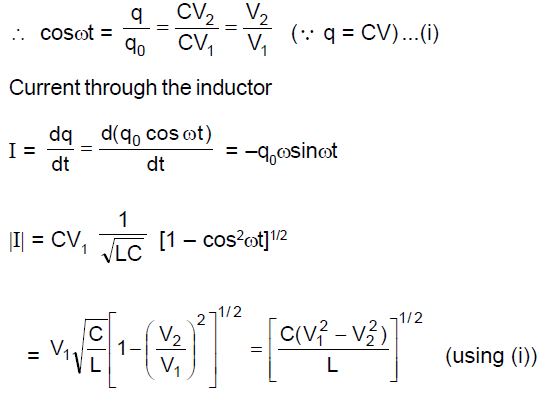•   Question 22

The dependence of acceleration due to gravity g on the distance r from the centre of the earth, assumed to be a sphere of radius R of uniform density is as shown in figures below. The correct figure is.

A :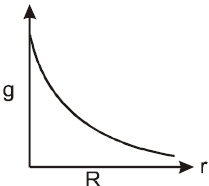B :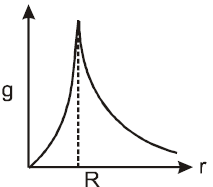C :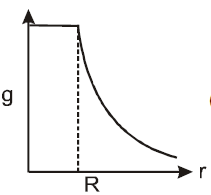D :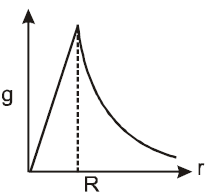•
.

Solution :
The acceleration due to gravity at a depth d below surface of earth is
g'=GM/R2 (1 - d/R) = g(1 - d/R)
g' = 0 at d = R acceleration due to gravity is zero at the centre of earth . Thus, the variation in value g with r is for, r > R,
g' ∝ 1/r2
Here, R + h =r
rg'=gr/R
here R-d = r
g' ∝ r
Therefore, the variation of g with distance from centre of the earth will be as shown in the figure.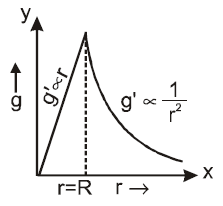•   Question 23

Asolid cylinder and a hollow cylinder, both of the samemass and same external diameter are relaeased from the same height at the same time on an inclined plane. Both roll down without slipping.Which one will reach the bottom first ?

A : Both together only when angle of inclination of plane is 45°
B : Both together
C : Hollow cylinder
D : Solid cylinder

•
.

Solution :
time taken to reach the bottom of inclined plane
t = √(2l(1 + K2/R2)/gsinΘ)
here l is the length of the incline plane
For solid cylinder K2 =R2/2
For Hollow cylinder = K2 =R2
Hence, solid cylinder will reach the bottom first.

•   Question 24

The thermo e.m.f. E in volts of a certain thermocouple is found to vary with temperature difference Θ in °C between the two junctions according to the relation
E = 30Θ - Θ2/15
The neutral temperature for the thermo-couple will be

A : 450°
B : 400°
C : 225°
D : 30°

•
.

Solution :
E = 30Θ - Θ2/15
dE/dΘ = 0;
dE/dΘ = 30 -2Θ/15
Θ=15*30/2 =225°C

•   Question 25

(i) Centre of gravity (C.G.) of a body is the point at which the weight of the body acts
(ii) Centre of mass coincides with the centre of gravity if the earth is assumed to have infinitely large radius
(iii) To evaluate the gravitational field intensity due to any body at an external point, the entire mass of the body can be considered to be concentrated at its C.G.
(iv) The radius of gyration of any body rotating about an axis is the length of the perpendicular dropped from the C.G. of the body to the axis.
Which one of the following pairs of statements is correct ?

A : iv and i
B : i and ii
C : ii and iii
D : iii and iv

•
.

•   Question 26

The magnetic moment of a diamagnetic atom is

A : much greater than one
B : one
C : between zero and one
D : equal to zero

•
.

Solution :
The magnetic momentum of a diamagnetic atom is equal to zero.

•   Question 27

Two identical bar magnets are fixed with their centres at a distance d apart. a stationary charge Q is placed at P in between the gap of the two magnets at a distance D from the centre O as shown in the figure
The force on the charge Q is?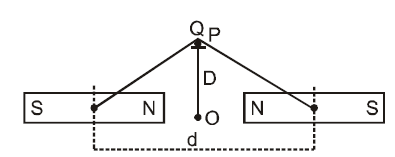A : Zero
B : directed along OP
C : directed along PO
D : directed perpendicular to the plane of paper

•
.

Solution :
Magnetic field due to bar magnets exerts force on moving charges only. Since the charge is at rest, zero force acts on it.

•   Question 28

Aparticle ofmass M, starting from rest, undergoes uniform acceleration. If the speed acquired in time T is V, the power delivered to the particle is

A : MV2/T
B : 1/2 * MV2/T2
C : MV2/T2
D : 1/2 * MV2/T

•
.

Solution :
Power delivered in time T is
P = F.V. = MaV
P = MVdV/dT
PT=MV2/2

•   Question 29

A thin circular ring of mass M and radius r is rotating about its axis with constant angular velocity ?. Two objects each of mass m are attached gently to the opposite ends of a diameter of the ring. The ring now rotates with angular velocity given by

A : (M + 2m)w/2m
B : 2Mw/(M+2m)
C : (M+2m)w/M
D : Mw/(M+2m)

•
.

Solution :
As no external toruqe is acting about the axis, angular momentum of system remains conserved.
I1w1 = I2w2
w2 = Mw/(M+2m)

•   Question 30

A monoatomic gas at pressure P1 and volume V1 is compressed adiabatically to 1/8th of its original volume. What is the final pressure of the gas

A : 64P1
B : P1
C : 16P1
D : 32P1

•
.

Solution :
Ideal gas equation, for an adiabatic process is
PVγ = constant
P1V1γ =P2V2γ
For monoatomic gas γ =5/3;
P1V15/3 = P2(V1/8 5/3)

•   Question 31

Among the elements Ca, Mg, P and Cl, the order of increasing atomic radii is :

A : Mg < Ca < Cl < P
B : Cl < P < Mg < Ca
C : P < Cl < Ca < Mg
D : Ca < Mg < P < Cl

•
.

Solution :
The atomic radii decreases on moving from left to right in a period, thus order of sizes for Cl, P and Mg is Cl < P < Mg. Down the group size increases. Thus overall order is : Cl < P < Mg < Ca.

•   Question 32

The reaction,is begun with the concentrations of A and B both at an initial value of 1.00 M. When equilibrium is reached, the concentration of D is measured and found to be 0.25 M. The value for the equilibrium constant for this reaction is given by the expression :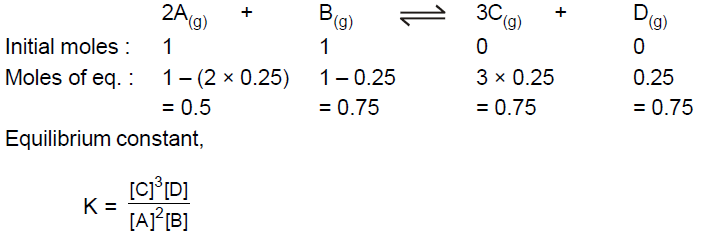A : [(0.75)3 (0.25)] / [(1.00)2 (1.00)]
B : [(0.75)3 (0.25)] /[(0.50)2 (0.75)]
C : [(0.75)3 (0.25)] / [(0.50)2 (0.25)]
D : [(0.75)3 (0.25)] / [(0.75)2 (0.25)]

•
.•   Question 33

Which of the following expressions correctly represents the equivalent conductance at infinite dilution of Al2(SO4)3. Given that ÅAl3+ and ÅSO42- are the equivalent conductances at infinite dilution of the respective ions?

A : Al3+ + 3ÅSO42-
B : ÅAl3+ + ÅSO42-
C : Al3+ + 3ÅSO42-) * 6
D : 1/3 ÅAl3 + 1/2 + ÅSO42-

•
.

Solution :
At infinite dilution, when dissociation is complete, each ion makes a definite contribution towards molar conductance of the electrolyte irrespective of the nature of the other ion with which it is associated

•   Question 34

The pressure exerted by 6.0 g of methane gas in a 0.03m3 vessel at 129° C is (Atomic masses) : C = 12.01, H = 1.01 and R = 8.314 J K-?1 mol?-1) :

A : 215216Pa
B : 13409Pa
C : 41648Pa
D : 31684Pa

•
.

Solution :
T = 129°C = 129 + 273 = 402 K
V = 0.03m3
Molecular mass of CH4, M = 12.01 + 4 * 1.01 = 16.05
PV = nRT = RTw/M
P=41647.7 Pa

•   Question 35

Match List I (Equations) with List II (Types of processes) and select the correct option.
 List 1 Equations List II Types of process a)Kp > Q i)Non-spontaneous b)ΔG° < RT In Q ii)Equilibrium c)Kp = Q iii)Spontaneous and endothermic d)T = ΔH/ΔS vi)Spontaneous

A : a - (i), b - (ii), c - (iii), d - (iv)
B : a - (iii), b - (iv), c - (ii), d - (i)
C : a - (iv), (b -(i), c - (ii), d - (iii)
D : a - (ii), b - (i), c - (iv), d - (iii)

•
.

Solution :
When Kp > Q, rate of forward reaction > rate of backward reaction.
Reaction is spontaneous. When ΔG° < RT ln Q, ΔG° is positive, reverse reaction is feasible, thus reaction is non-spontaneous.
When Kp = Q, rate of forward reaction = rate of backward reaction
Reaction is in equilibrium. When TΔS > ΔH, ΔG will be negative only when ΔH = + ve

•   Question 36

Among the following four compounds :
(i) Phenol
(ii) Methyl phenol
(iii)Meta-nitrophenol
(iv) Para-nitrophenol
The acidity order is :

A : (iv) > (iii) > (i) > (ii)
B : (iii) > (iv) > (i) > (ii)
C : (i) > (iv) > (iii) > (ii)
D : (ii) > (i) > (iii) > (iv)

•
.

Solution :
In phenols, the presence of electron releasing groups decreases the acidity, whereas presence of electron withdrawing groups increase the acidity, compared to phenol. Among the meta and para-nitrophenols, the latter is more acidic as the present of -NO2 group at para position stabilises the phenoxide ion to a greater extent than when it is present at meta position.

•   Question 37

Among the following which one has the highest cation to anion size ratio?

A : CsI
B : CsF
C : LiF
D : NaF

•
.

Solution :
The order of size of given cations is Li+ < Na+ < Cs+ and the order of size of given anions is I?- > F-? Thus, when the cation is large and anion is smallest, the cation to anion size ratio is maximum. Hence, cation to anion size ratio is maximum for CsF.

•   Question 38

Three moles of an ideal gas expanded spontaneously into vacuum. The work done will be :

A : Infinite
B : 3 Joules
C : 9 Joules
D : Zero

•
.

Solution :
Since the ideal gas expands spontaneously into vacuum, Pext = 0, hence work done is also zero.

•   Question 39

Which of the following species is not electrophilic in nature?

A :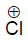B :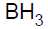C :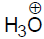D :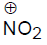•
.

Solution :
Electrophiles are electron deficient species.Among the given, 3) has lone pair of electrons for donation, thus it is not electron deficient and hence, does not behave like an electrophile.

•   Question 40

A 0.66 kg ball is moving with a speed of 100 m/s. The associated wavelength will be : (h = 6.6 * 10-?34 Js)

A : 6.6 * 10-?32
B : 6.6 * 10-?34
C : 1.0 * 10-?35
D : 1.0 * 10-?32

•
.

Solution :
use de-Broglie equation, λ = h/mv

•   Question 41

Which of the following is not a fat soluble vitamin ?

•
.

Solution :
Vitamin B complex is fat insoluble

•   Question 42

Which of the statements about "Denaturation" given below are correct ?
Statements:
(a) Denaturation of proteins causes loss of secondary and tertiary structures of the protein.
(b) Denturation leads to the conversion of double strand of DNA into single strand
(c) Denaturation affects primary strucrture which gets distorted

•
.

Solution :
During denaturation secondary and tertiary structures of protein destroyed but primary structures remains intact.

•   Question 43

Which has the maximum number of molecules among the following ?

•
.

Solution :
Moles of 1: 44/44 =1 →so number of molecules = Na
Moles of 2: 48/48 =1 →so number of molecules = Na
Moles of 3: 8/2=4 →so number of molecules = 4Na Moles of 4: 64/64 =1 →so number of molecules = Na

•   Question 44

The half life of a substance in a certain eznzyme-catalysed reaction is 138 s. The time required for the concentration of the substance to fall from 1.28 mg L-1 to 0.04 mg L-1 ,is:

•
.

Solution :
Enzyme catalysed reactions are initially follow first order kinetics when concentration decreases 1.28 mg L-1to 0.04 mg L-1 .Then five half life completed
No. of half lives = 5
So, times required = 5 � 138 = 690 s

•   Question 45

Which of the following compounds undergoes nucleophilic substitution reaction most easily ?

•
.

Solution :
The correct order of nucleophilic substitution reactions
A>D>B>C

•   Question 46

Which of the following statements is incorrect ?

•
.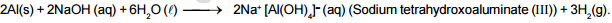•   Question 47

A 0.1 molal aqueous solution of a weak acid is 30% ionized. If Kf for water is 1.86°C/m, the freezing point of the solution will be :

•
.

Solution :
ΔTf = iKfm
HA → H+ + AH-
1-α → α α
1-0.3 → 0.3 0.3
i = 1 - 0.3 + 0.3 + 0.3
i = 1.3
ΔTf = 1.3 *1.86 *0.1 = 0.2418
Tf = 0 - 0.2418 = -0.2418°C

•   Question 48

The rate of the reaction 2N2O5 → 4NO2 + O2 can be written in three ways :

-d[N2O5]/dt = k[N2O5]

d[NO2]/dt = k'[N2O5]

d[O2 ]/dt = k"[N2O5]

The relationship between k and k' and between k and k" are :

•
.

Solution :
-1/2 d[N2O5]/dt = 1/4 d[NO2]/dt = d[O2 ]/dt

1/2 k[N2O5] = 1/4 k'[N2O5] = k"[N2O5]

•   Question 49

Which of the following carbonyls will have the strongest C-O bond ?

•
.

Solution :
As + ve charge on the central metal atom increases, the less readily the metal can donate electron density into the π* orbitals of CO ligand to weaken the C-O bond. Hence the C-O bond would be strongest in Mn(CO)6+

•   Question 50

The order of reactivity of phenyl magnesium bromide (PhMgBr) with the following compounds :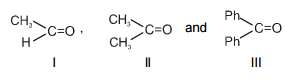•
.

Solution :
4 due to steric crowding

•   Question 51

The IUPAC name of the following compound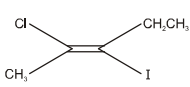•
.

•   Question 52

According to the Bohr Theory, which of the following transitions in the hydrogen atom will give rise to the least energetic photon ?

•
.

Solution :
Energy of photon obtained from the transition n = 6 to n = 5 will have least energy.
ΔE = 13.6Z2(1/n12 - 1/n22)

•   Question 53

A solid compound XY has NaCl structure. If the radius of the cation is 100 pm, the radius of the anion (Y-) will be :

•
.

Solution :
Radius ratio of NaCl like crystal = r+ / r- = 0.414
r- = 100 / 0.414 =241.5

•   Question 54

Consider the following processes and their ΔH((kJ/mol) :
1/2 A → B (+150)
3B → 2C + D -(125)
E + A → 2D (+350)
For B + D → E + 2C, ΔH will be

•
.

Solution :
by doing 2*eq(1) + eq(2) - eq(3) we will get the asked equation
ΔH = 300 -125 -350 =-175

•   Question 55

Match the compounds given in List-I with List-II and select the suitable option using the code given below :
 List1 List2 a)Benzaldehyde i)Phenolphthalein b)Phthalic anhydride ii)Benzoin condensation c)Phenyl benzoate iii)Oil of wintergreen d)Methyl salicylate (iv) Fries rearrangement

a,b,c and d are

•
.

•   Question 56

Which of the following compounds is most basic ?

•
.

Solution :
In benzylamine, the lone pair on nitrogen is not delocalized with the benzene ring.

•   Question 57

Which of the following structures is the most preferred and hence of lowest energy for SO3 ?

•
.

Solution :
Formal charges help in the selection of the lowest energy structure from a number of possible Lewis structures for a given species. Generally the lowest energy structure is the one with the smallest formal charges on the atoms.

•   Question 58

A solution contains Fe2+, Fe3+ and I- ions. This solution was treated with iodine at 35°C. E° for Fe3+/Fe2+ is + 0.77 V and E° for I2/2I- = 0.536 V. The favourable redox reaction is

•
.

Solution :
Since the reduction potential of Fe3+/Fe2+ is greater than that of I2/2I- , Fe3+ will be reduced and I- will be oxidized

•   Question 59

What is the value of electron gain enthalpy of Na+ if IE1 of Na = 5.1 eV ?

•
.

Solution :
Electron gain enthalpy is the ΔH for the process
Na+ + e- → Na
ΔH = -5.1eV

•   Question 60

The unit of rate constant for a zero order reaction is :

•
.

•   Question 61

In qualitative analysis, the metals of Group I can be separated from other ions by precipitating them as chloride slats. A solution initially contains Ag+ and Pb2+ at a concentration of 0.10 M. Aqueous HCl is added to this solution until the Cl- concentration is 0.10 M. What will be concentrations of Ag+ and Pb2+ be at equilibrium?
(Ksp for AgCl = 1.8 * 10-10, Ksp for PbCl2 = 1.7 * 10�5)

•
.

Solution :
[Ag+] = 1.8 * 10-10/0.1

[Pb2+] = 1.7 * 10-5/ (0.1)2

•   Question 62

A bubble of air is underwater at temperature 15°C and the pressure 1.5 bar. If the bubble rises to the surface where the temperature is 25°C and the pressure is 1.0 bar, what will happen to the volume of the bubble?

•
.

Solution :
V ∝ T/P
V2 ∝ 298
V1 ∝ 288/1.5
V2 /V1 = 1.55

•   Question 63

Match List-I with List-II for the compositions of substances and select the correct answer using the code given below the lists :
CaSO4.1/2H2O
 Substances Composition a)Palster of paris i)CaSO4.2H2O b)Epsomite ii) c)kieserite iii)MaSO4.7H2O d)Gypsum (iv)MaSO4.H2O (v)CaSO4

•
.

•   Question 64

The pairs of species of oxygen and their magnetic behaviours are noted below. Which of the following presents the correct description ?

•
.

Solution :
they contain one and two unpaired electrons each.

•   Question 65

Consider the reactions :
The mechanisms of reactions (i) and (ii) are respectively :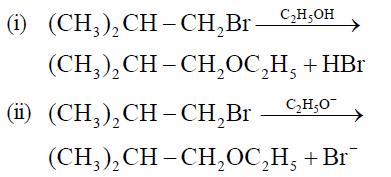•
.

Solution :
Rearrangements do not occur in the given substitution reactions. So carbocation intermediates are not involved. Hence the mechanism is SN2 in both cases.

•   Question 66

Which of the following complex compounds will exhibit highest paramagnetic behaviour ?
(At. No. Ti 22, Cr = 24, Co = 27, Zn = 30)

•
.

Solution :
[Cr(NH3)6]3+ contain three unpaired electrons.

•   Question 67

200mL of an aqueous solution of a protein contains its 1.26 g. The Osmotic pressure of this solution at 300K is found to be 2.57 * 10-?3 bar. The molar mass of protein will be (R = 0.083 L bar mol?-?1 K-?1) :

•
.

Solution :
πv=nST
M = W*S*T/π*v

•   Question 68

Which of the following oxide is amphoteric ?

•
.

Solution :
because it reacts with acids as well as bases to form corresponding salts.

•   Question 69

The following reactions take place in the blast furnace in the preparation of impure iron. Identify the reaction pertaining to the formation of the slag.

•
.

Solution :
Slag can be defined as a fusiblemass, which is obtained when a flux reacts with an infusible acidic or basic impurity present in the oxide ore.
CaO(s) (basic flux) + SiO2 (s) (acidic flux) → CaSiO3 (s) (slag)

•   Question 70

An organic compound ?'A'? on treatment with NH3 gives ?'B'? which on heating gives ?'C?', ?'C?' when treated with Br2 in the presence of KOH produces ethylamine. Compound ?'A'? is :

•
.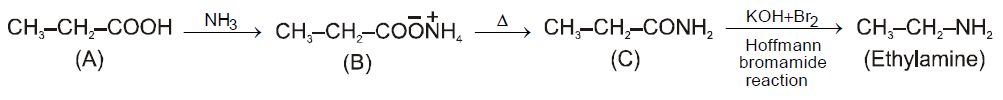•   Question 71

The technique called gamete intrafallopian transfer (GIFT) is recommended for those females:

•
.

•   Question 72

Which one of the following is a possibility for most of us in regard to breathing, by making a conscious effort?

•
.

Solution :
Eustachian tube connect middle ear cavity (Tympanic cavity) with pharynx

•   Question 73

Bacillus thuringiensis forms protein crystals which contain insecticidal protein

•
.

•   Question 74

Which one of the following pairs is wrongly matched while the remaining three are correct?

•
.

Solution :
Water hyacinth is offset.

•   Question 75

Which one of the following diagrams represents the placentation in Dianthus?

•
.

Solution :
Free central placentation occurs in Dianthus

•   Question 76

Which one of the following statements is totally wrong about the occurrence of notochord, while the other three are correct?

•
.

Solution :
Because get changed or replaced by vertibral column

•   Question 77

Which one of the following animals may occupy more than one trophic levels in the same ecosystem at the same time?

•
.

Solution :
It feeds upon grains hence called primary consumer and also insects hence called secondary consumer

•   Question 78

Both, hydrarch and xerarch successions lead to:

•
.

•   Question 79

What happens during fertilisation in humans after many sperms reach close to the ovum?

•
.

•   Question 80

About which day in a normal human menstrual cycle does rapid secretion of LH (Popularly called LH-surge) normally occurs?

•
.

•   Question 81

The damping force on a oscillator is directly proportional to the velocity. The Unit of the constant of proportionality are

A : kgs-1
B : kgs
C : kgms-1
D : kgms-2

•
.

Solution :
F= Kv
Unit of K = kgms-2 /ms-1

•   Question 82

The motion of a particle along a straight line is described by equation
x = 8 + 12t - t3
where x is in meter and t in second. The retardation of the particle when its velocity becomes zero is

A : 6 ms-2
B : 12 ms-2
C : 24 ms-2
D : 0

•
.

Solution :
x = 8 + 12t - t3
v = dx/dt = 12 - 3t2
a = dv/dt = -6t
putting v = 0
3t2 = 12
t = 2 sec
thus a = -6*2 = -12
retardation of 12 ms-2

•   Question 83

The horizontal range and the maximum height of a projectile are equal . The angle of projection of the projectile is:

A : tan-1(2)
B : 45°
C : tan-1(1/4)
D : tan-1(4)

•
.

Solution :
tan Θ = 4H/R
given H = R

•   Question 84

A particle has initial velocity (2ĩ + 3j̃) and acceleration (0.3ĩ + 0.2j̃).The magnitude of velocity after 10 seconds will be

A : 5 units
B : 9 units
C : 9√2
D : 5√2

•
.

Solution :
v = u + at
v = (2ĩ + 3j̃)+(0.3ĩ + 0.2j̃)*10
ṽ = 5ĩ + 5j̃
v = 5√2 units

•   Question 85

A car of mass 1000 kg negotiates a banked curve of radius 90m on a frictionless road. If the banking angle is 45°, the speed of the car is

A : 5 ms-1
B : 10 ms-1
C : 20 ms-1
D : 30 ms-1

•
.

Solution :
v = √(rg tanΘ)
v = √(90*10*tan45°)
=30

•   Question 86

A solid cylinder of mass 3 kg is rolling on a horizontal surface with velocity 4 ms-1. It collides with a horizontal spring of force constant 200 Nm-1 . The maximum compression produced in the spring will be

A : 0.7m
B : 0.2m
C : 0.5m
D : 0.6m

•
.

Solution :
1/2 mv2 [1 + K2/R2]

1/2kx2
putting m = 3kg, v = 4 m/s,
K2/R2 = 1/2 (For solid cylinder), k = 200 Nm-1 we get
xmax = 0.6m

•   Question 87

The potential energy of a particle in a force field is :
U = A/r2 - B/r , where A and B are positive constants and r is the distance of particle from the centre of the field. For stable equilibrium, the distance of the particle is

A : A/B
B : B/A
C : B/2A
D : 2A/B

•
.

Solution :
For Stable equilibrium, F = -dU/dr = 0
we get r = 2A/B

•   Question 88

Two spheres A and B of masses m1 and m2 respectively collide. A is at rest initially and B is moving with velocity v along x-axis. After collision B has a velocity v/2 in a direction perpendicular to the original direction. The mass A moves after collision in the direction.

A : Θ = tan-1(1/2) to the x-axis
B : Θ = tan-1(-1/2) to the x-axis
C : same as that of B
D : opposite to the of B

•
.

Solution :
Applying the law of conservation of momentum we have
in y-direction, m1 u sin Θ = m2 v/2 .....(i)
In x-direction, m1 u cos Θ = m2 v .....(ii)

•   Question 89

Two persons of masses 55 kg and 65kg respectively, are at the opposite ends of a boat. The length of the boat is 30. m and weighs 100 kg. The 55 kg man walks up to the 65 kg man and sits with him. If the boat is in still water the center of mass of the system shift by:

A : zero
B : 0.75m
C : 3.0m
D : 2.3m

•
.

Solution :
Since net external force on the system is zero hence C.O.M. remains unchanged

•   Question 90

ABC is an equilateral triangle with O as its centre F1,F2 and F3 represent three forces acting along the sides AB, BC and AC respectively. If the torque about O is zero then the magnitude of F3 is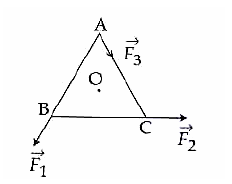A : (F1+F2)/2
B : 2(F1+F2)
C : F1+F2
D : F1-F2

•
.

Solution :
Taking anticlockwise torque
F1d + F2d - F3d = 0 (where d = perpendicular distance of the centre O from each side)
F3 = F1 + F2

•   Question 91

When a mass is rotating in a plane about a fixed point, its angular momentum is directed along

B : the tangent to the orbit
C : a line perpendicular to the plane of rotation
D : the line making an angle of 45° to the plane of rotation.

•
.

Solution :
L=r x mv

•   Question 92

A spherical planet has a mass MP and diameter DP. A particle of mass m falling freely near the surface of this planet will experience an acceleration due to gravity, equal to

A : GMP/ DP2
B : 4GMPm/ DP2
C : 4GMP/ DP2
D : GMPm/ DP2

•
.

Solution :
g = GMP/ RP2
where RP = DP/2

•   Question 93

A geostationary satellite is orbiting the earth at a height of 5R above that surface of the earth, R being the radius of the earth. The time period of another satellite of the earth. The time period of another satellite in hours at a height of 2R from the surface of the earth is

A : 6√2
B : 6/√2
C : 5
D : 10

•
.

Solution :
According to Kepler's third law, T2 ∝ r3
T2/T1 = (r2/r1)3/2
Given T1 = 24 h r1 = R + h1 = R + 5R = 6R
r2 = R + h2 = R + 2R = 3R

On solving we get 6√2

•   Question 94

The height at which the weight of a body becomes 1/16th , its weight on the surface of earth (radius R), is

A : 3R
B : 4R
C : 5R
D : 15R

•
.

Solution :
Wh/W = mg/mg = (R/(R+h))2 = 1/16
h=3R

•   Question 95

Two sources of sound placed close to each other, are emitting progressive waves given by y1 = 4 sin 600 πt and y2 = 5 sin 608 πt: An observer located near these two sources of sound will hear

A : 8 beats per second with intensity ratio 81 : 1 between waxing and waning
B : 4 beats per second with intensity ratio 81 : 1 between waxing and waning
C : 4 beats per second with intensity ratio 25 : 16 between waxing and waning
D : 8 beats per second with intensity ratio 25 : 16 between waxing and waning

•
.

Solution :
Comapare both equations with y = a sin 2πft we get
f1=300Hz
f2=304Hz
Number of beats = f2-f1 = 4s-1
Imax/Imin = ((a1 + a2)/(a1 - a2))
((4+5)/(4-5))2 = 81/1

•   Question 96

When a string is divided into three segments of length l1, l2 and l3, the fundamental frequencies of these three segments are v1, v2 and v3 respectively. The original functamental frequency (v) of the string is :

A : 1/v = 1/v1 + 1/v2 + 1/v3
B : 1/√v = 1/√v1 +1/√v2 +1/√v3
C : √v = √v1 + √v2 + √v3
D : v = v1 +v2 +v3

•
.

Solution :
Length of the string l = l1 + l2 + l3 v ∝ 1/l

•   Question 97

One mole of an ideal gas goes from an initial state A to final state B via two processes : It first undergoes isothermal expansion from volume V to 3V and then its volume is reduced from 3V to V at constant pressure. The correct P-V diagram representing the two processes is:

A :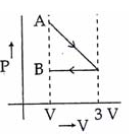B :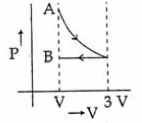C :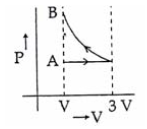D :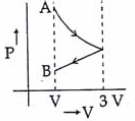•
.

Solution :
For isothermal expansion P-V curve is rectangular hyperbola (clockwise curve)

•   Question 98

A thermodynamics system is taken trough the cycle ABCD as shown in figure. Heat rejected by the gas during the cycle is: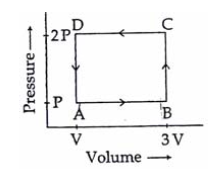A : 1/2 PV
B : PV
C : 2PV
D : 4PV

•
.

Solution :
For given cyclic process, ΔU = 0
Q = W (From first law of thermodynamic Q = ΔU + W) Also W = - area enclosed by the curve = - P * 2V
Heat rejected = 2PV

•   Question 99

Liquid oxygen at 50 K is heated to 300 K at constant pressure of 1 atm. The rate of heating is constant. Which one of the following graphs represents the variation of temperature with time ?

A :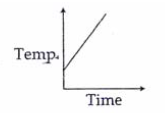B :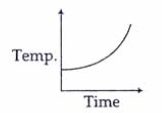C :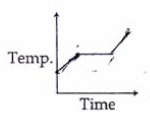D :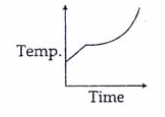•
.

Solution :
At first temperature will increase then there will be state change from liquid to gas.

•   Question 100

If the radius of a star is R and it acts as a black body, what would be the temperature of the star, in which the rate of energy production is Q ?(&sigma is Stefan's constant)

A : (4πR2Q/σ)1/4
B : (Q/4πR2Qσ)1/4
C : Q/4πR2σ
D : (Q/4πR2Qσ)-1/2

•
.

Solution :
Using Stefan's law, the rate of energy production is Q = E * A

•   Question 101

A coil of resistance 400Ω is placed in a magnetic filed. if the magnetic flux φ (wb) linked with the coil varies with times t(sec) as
φ = 50t2 + 4
The current is the coil at t = 2 sec is

A : 2A
B : 1A
C : 0.5A
D : 0.1A

•
.

Solution :
i = |(-dφ/dt)/R| = 0.5A

•   Question 102

The current (I) in the inductance is varying with time according to the plot shown in figure.
Which one of the following is the correct variation of voltage with time in the coil ?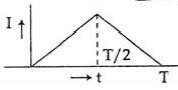A :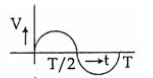B :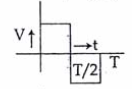C :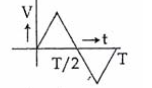D :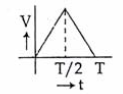•
.

Solution :
|V|=|-Ldi/dt| therefore answer will be best represented by graph -2

•   Question 103

In an electrical circuit R, L, C and an a.c. voltage source are all connected in series. When L is removed from the circuit, the phase difference between the voltage and the current in the ciruit is π/3 . If instead, C is removed from the circuit, the phase difference is again π/3 . The power factor of the circuit is

A : 1
B : √3/2
C : 1/2
D : 1/√2

•
.

Solution :
It is the condition of resonance therefore phase difference between voltage and current is zero and power factor cos &phi = 1

•   Question 104

A ring is made of a wire having a resistance R0 = 12Ω. Find the points A and B, as shown in the figure, at which a current carrying conductor should be connected so that the resistance R of the sub circuit between these points is equal to 8/3Ω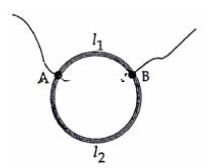A : l1/l2 = 3/8
B : l1/l2 = 1/2
C : l1/l2 = 5/8
D : l1/l2 = 1/3

•
.

Solution :
Since R∝l
According to problem R1 + R2 = 12Ω and (R1 * R2)/(R1 + R2) = 8/3Ω
On solving this we get R1 = 4Ω and R2 = 8Ω therefore the ratio l1/l2 =1/2

•   Question 105

If voltage across a bulb rated 220 Volts. 100 Watt drops by 2.5% of its rated value the percentage of the rated value by which the power would decrease is

A : 5%
B : 10%
C : 20%
D : 2.5%

•
.

Solution :
Power P = V2/R
For small variation,ΔP/P * 100% = 2*ΔV/V * 100%
therefore power would decrease by 5%

•   Question 106

In the circuit shown the cells A and B have negligible resistance. For VA = 12V, R1 = 500Ω and R = 100Ω the galvanometer (G) shows no deflection. The value of VB is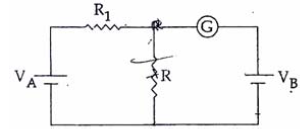A : 12V
B : 6V
C : 4V
D : 2V

•
.

Solution :
By using kirchoff voltage law the potential difference across R is 2 volt

•   Question 107

The electric field associated with an e. m. wave in vacuum is given by E = î 40 cos (kz � 6 � 108t) , where E, z and t are in volt/m, meter and seconds respectively. The value of wave vector k is :

A : 6 m-1
B : 3 m-1
C : 2 m-1
D : 0.5 m-1

•
.

Solution :
Equation of Electromagnetic wave is E = E0 cos (kz - ω t) and speed of EM wave V = ω/k
By comparing k = ω/v = 6 * 10 8/3* 10 8

•   Question 108

What is the flux through a cube of side 'a' if a point charge of q is at one of its corner

A : q/ε0
B : (q/2ε0) * 6a2
C : 2q/ε0
D : q/8ε0

•
.

•   Question 109

An electric dipole of moment 'p' is placed in an electric field of intensity 'E'. The dipole acquires a position such that the axis of the dipole makes an angle θ with the direction of the field. Assuming that the potential energy of the dipole to be zero when θ = 90°, the torque and the potential energy of the dipole will respectively be

A : pEsinθ , 2pEcosθ
B : pEcosθ,-pEsinθ
C : pEsinθ,-pEcosθ
D : pEsinθ,-2pEcosθ

•
.

Solution :
Toque = PEsinθ and Potential energy U = -PEcosθ

•   Question 110

Four point charge -Q, -q, 2q and 2Q are placed, one at each corner of the square. The relation between Q and q for which the potential at the centre of the square is zero is

A : Q = q
B : Q = 1/q
C : Q = -q
D : Q = -1/q

•
.

Solution :
Potential V at centre of the square =
-kQ/r - kq/r + k2Q/r + k2q/r = 0
So Q = -q

•   Question 111

A compass needle which is allowed to move in a horizontal plane is taken to a geomagnetic pole. It.

A : Will stay in north-south direction only
B : Will stay in east-west direction only
C : Will becomes rigid showing no movement
D : Will stay in any position

•
.

Solution :
Will remain in any position at geomagnetic north and south pole

•   Question 112

A milli voltmeter of 25 milli volt range is to be converted into an ammeter of 25 ampere rage. The value (in ohm) of necessary shunt will be

A : 1
B : 0.05
C : 0.001
D : 0.01

•
.

Solution :
If the resistance of the meter is G then the full scale deflection current ig = 25mV/G ampere

The Value of shunt required for converting it into ammeter of range 25 ampere is S = igG/i-ig
so 25mV/25

•   Question 113

Two similar coils of radius R are lying concentrically with their planes at right angles to each other. The currents flowing in them are I and 2I, respectively. The resultant magnetic field induction at the centre will be

A : µ0I/2R
B : µ0I/R
C : √5µ0I/2R
D : 0I/2R

•
.

Solution :
Magnetic field at the centre of circular current carrying coil of radius R and current I is =
µ0I/2R = B
Magnetic field at the centre of circular current carrying coil of radius R and current 2I is =
µ02I/2R = 2B
The resulting magnetic field will be =
√(B2+4B2)=√5B

•   Question 114

An alternating electric field, of frequency v, is applied across the dees (radius = R) of a cyclotron that is being used to accelerated protons (mass = m). The operating magnetic field (B) used in the cyclotron and the kinetic energy (K) of the proton beam, produced by it, are given by

A : B = 2πmv/e and K = 2mπ2v2R2
B : B = mv/e and K = m2πvR2
C : B = mv/e and K = 2mπ2v2R
D : B = 2πmv/e and K = m2πvR2

•
.

Solution :
frequency v = eB/2πm so B = 2πmv/e
Kinetic energy K = 1/2 mv2

•   Question 115

The magnifying power of a teloscope is 9. When it is adjusted for parallel rays the distance between the objective and eyepiece is 20 cm. The focal length of lenses are

A : 18 cm, 2 cm
B : 11 cm, 9 cm
C : 10 cm, 10 cm
D : 15 cm, 5 cm

•
.

Solution :
L = fo + fe = 20cm
M = fo/fe = 9

•   Question 116

A ray of light is incident at an angle of incidence, i, on one face of a prism of angle A (assumed to be small) and emerges normally from the opposite face. It the refractive index of the prism is µ, the angle of incidence i, is nearly equal to

A : A/µ
B : A/2µ
C : µA
D : µA/2

•
.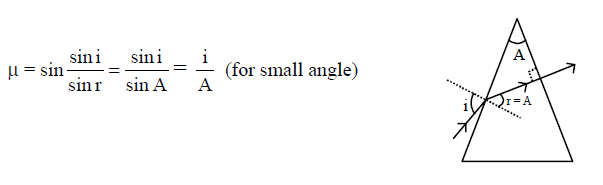•   Question 117

A concave mirror of focal length 'f1' is placed at a distance of 'd' from a convex lens of focal length 'f2' A beam of light coming from infinity and falling on this convex lens- concave mirror combination returns to infinity. The dsitance 'd' must equal

A : 2f1 + f2
B : -2f1 + f2
C : f1 + f2
D : -f1 + f2

•
.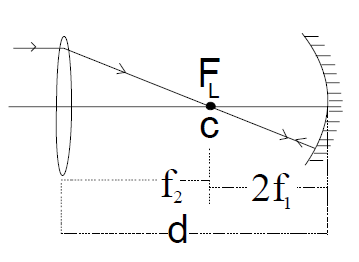•   Question 118

When a biconves lens of glass having refractive index 1.47 is dipped in a liqud, it acts as a plane sheet of glass. This implies that the liquid must have refractive index

A : greater than that of glass
B : less than that of glass
C : equal to that of glass
D : less than one

•
.

Solution :
1/f = (µ21 - 1)(1/R1 - 1/R2)
For power = 1/f = 0,
µ2 = µ1

•   Question 119

An α-particle moves in a circular path of radius 0.83 cm in the presence of a magnetic field of 0.25 Wb/m2. The de-Broglie wavelength associated with the particle will be

A : 10Å
B : 0.01Å
C :
D : 0.1Å

•
.

Solution :
radius R = mv/qB and de- Broglie wavelength λ = h/mv on solving we get λ= 0.01Å

•   Question 120

Monochromatic radiation emitted when electron on hydrogen atom jumps from first excited to the ground state irradiates a photosensitive material. The stopping potential is measured to be 3.57 V. The threshold frequency of the material is

A : 1.6 * 1015 Hz
B : 2.5 * 1015 Hz
C : 4 * 1015 Hz
D : 5 * 1015 Hz

•
.